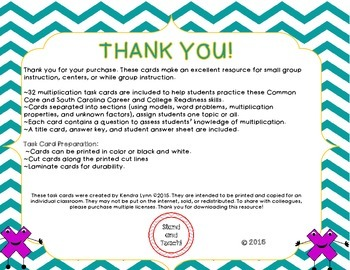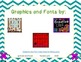Subject
Resource Type
Common Core State Standards
Product Rating
File Type

PDF (Acrobat) Document File

Be sure that you have an application to open this file type before downloading and/or purchasing.

1 MB|15 pages
Share
Product Description
Multiplication Task Cards: 32 task cards for use within your classroom! Provide students with meaningful engaging assignments based on their needs.

Common Core Standards:
3.OA.1 Interpret products of whole numbers, e.g., interpret 5 × 7 as the total number of objects in 5 groups of 7 objects each. For example, describe a context in which a total number of objects can be expressed as 5 × 7.

3.OA.3 Use multiplication and division within 100 to solve word problems in situations involving equal groups, arrays, and measurement quantities, e.g., by using drawings and equations with a symbol for the unknown number to represent the problem.1
3.OA.4 Determine the unknown whole number in a multiplication or division equation relating three whole numbers. For example, determine the unknown number that makes the equation true in each of the equations 8 × ? = 48, 5 = _ ÷ 3, 6 × 6 = ?

3.OA.5 Apply properties of operations as strategies to multiply and divide.2 Examples: If 6 × 4 = 24 is known, then 4 × 6 = 24 is also known. (Commutative property of multiplication.) 3 × 5 × 2 can be found by 3 × 5 = 15, then 15 × 2 = 30, or by 5 × 2 = 10, then 3 × 10 = 30. (Associative property of multiplication.) Knowing that 8 × 5 = 40 and 8 × 2 = 16, one can find 8 × 7 as 8 × (5 + 2) = (8 × 5) + (8 × 2) = 40 + 16 = 56. (Distributive property.)
3.OA.7 Fluently multiply and divide within 100, using strategies such as the relationship between multiplication and division (e.g., knowing that 8 × 5 = 40, one knows 40 ÷ 5 = 8) or properties of operations. By the end of Grade 3, know from memory all products of two one-digit numbers.

South Carolina College and Career Readiness Standards:
3.ATO.1 Use concrete objects, drawings and symbols to represent multiplication facts of two single-digit whole numbers and explain the relationship between the factors (i.e., 0 – 10) and the product.
3.ATO.3 Solve real-world problems involving equal groups, area/array, and number line models using basic multiplication and related division facts. Represent the problem situation using an equation with a symbol for the unknown.
3.ATO.4 Determine the unknown whole number in a multiplication or division equation relating three whole numbers when the unknown is a missing factor, product, dividend, divisor, or quotient.
3.ATO.5 Apply properties of operations (i.e., Commutative Property of Multiplication, Associative Property of Multiplication, Distributive Property) as strategies to multiply and divide and explain the reasoning.
3.ATO.7 Demonstrate fluency with basic multiplication and related division facts of products and dividends through 100.
Total Pages
15 pages
Included
Teaching Duration
N/A
Report this Resource to TpT
Reported resources will be reviewed by our team. Report this resource to let us know if this resource violates TpT’s content guidelines.